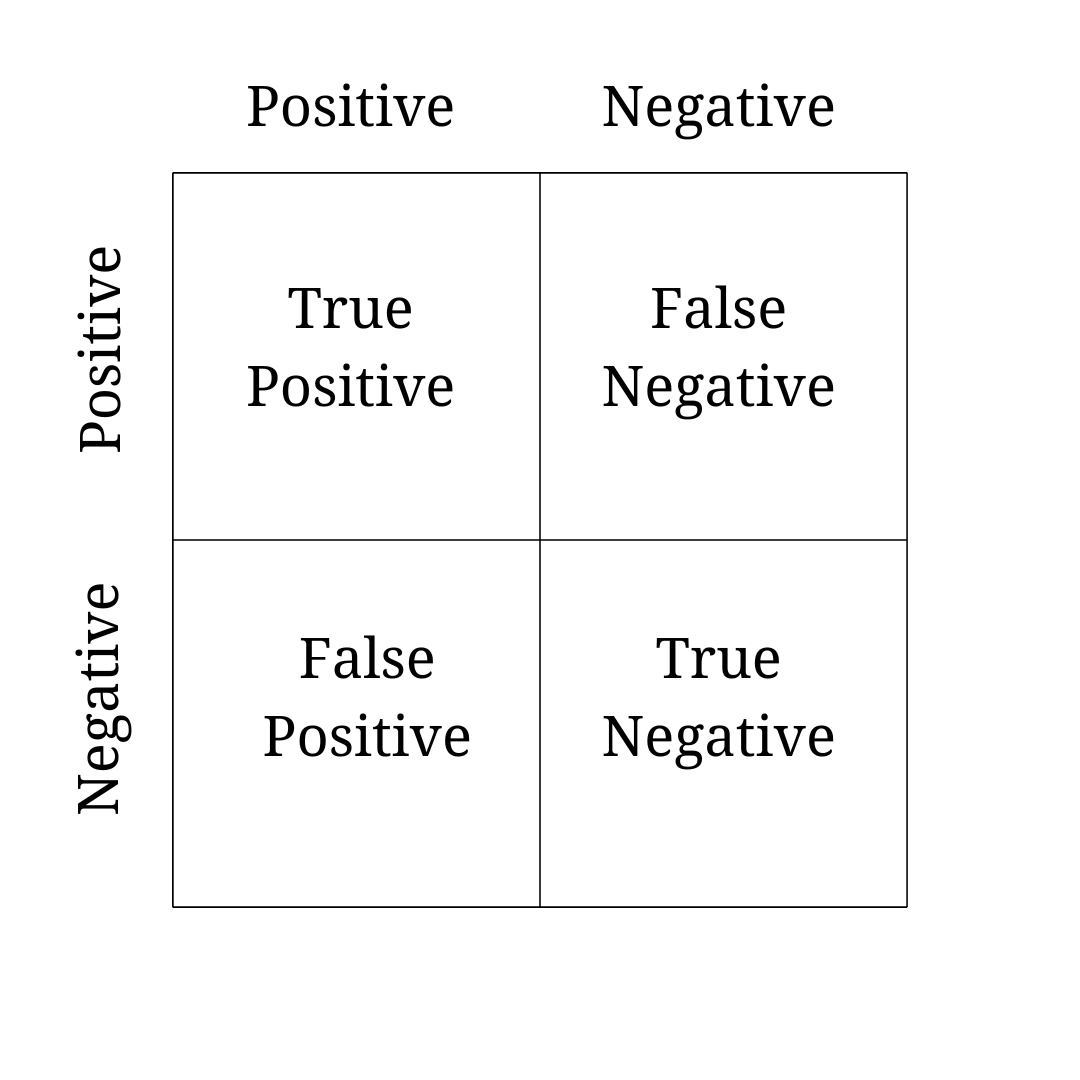# What is the definition of True Positive?

True Positive - the proportion of positives in a group that were assessed correctly.

• Test Results: Although most tests/assessments result in either a positive or negative, the accuracy of a test is dependent on the number of positives and negatives that are correct. Therefore, when the accuracy of a test is determined, there are 4 possible results, because a positive or negative result could be right (true) or wrong (false).## Related Terms

• True negative
• False positive
• False negative
• Sensitivity
• Specificity

## Synonyms

• Correct positive
• True hit
• True detection